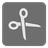Most Efficient Cutting
Elementary+
English

Metal strips, pipes, rods, etc. are being delivered in lengths of 6000 millimeters (6 meters). We want to cut them into pieces of different lengths.

Write a piece of code that finds how to do this in the most efficient way, that is, with the least possible quantity of material wasted. You are given the requested pipe lengths (as a list of integers). You should find the most efficient way of cutting, and return the sum of the wasted pipe length.

Input: A 'BOM' (Bill Of Materials), a list of lengths in millimeters that have to be cut (list of integer).

Output: The sum of wasted pipe length (Integer).

Example:

```most_efficient_cutting([3000, 2200, 2000, 1800, 1600, 1300]) == 100
most_efficient_cutting([4000, 4000, 4000]) == 6000
```

How it is used:
To find the most efficient way of using the given resources.

Precondition:
all(1 <= b <= 6000 for b in bom)